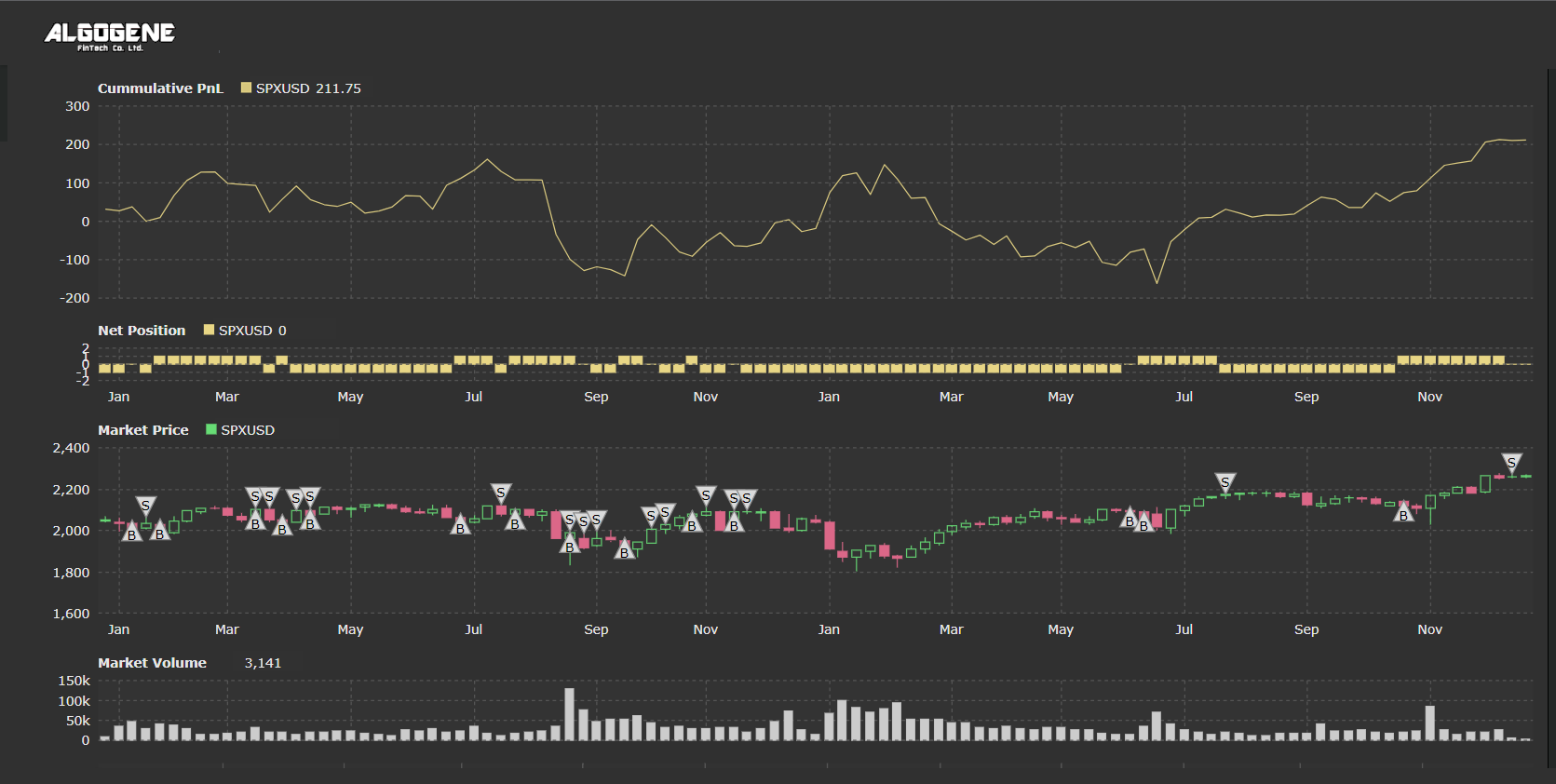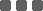## A trend following strategy based on volatility approach

In this article, we will introduce a simple trend following strategy using a volatility approach.

### Volatility Decomposition: Upside vs Downside

Volatility is used to reflect the magnitude of market fluctuation, and it is usually used to observe market sentiment or predict market trends. In financial market, due to the behavior of "Market take the stairs up and the elevator down", the volatility distribution is not symmetric. Therefore, it is necessary to distinguish upside and downside volatility.

In this article, we take the opening price as the benchmark, the fluctuation above the opening price is defined as the upward volatility, otherwise it is the downward volatility. Under normal circumstances, the upward volatility is greater than the downward volatility when the market is in the upward trend, and vice versa in the downward trend.

### Calculation

In above definition, the difference between upward and downward volatility is measured as:

difft := (Hight + Lowt ) / Opent - 2

To smooth out the short-term fluctuations, a N-day moving average is applied.

Smoothed Difft = (difft + difft-1 + difft-N+1 ) / N

The market is said to be in an upward trend when the Smoothed Difft is calculated to be positive; and vice versa in a downward trend when its value is negative.

Suppose we take a 60-day moving average, and apply this strategy to the daily closing price of SP500 Index CFD (i.e. SPXUSD) over the year of 2015.01 - 2016.12

• Based on a sliding window approach to collect the previous 250 closing price
• Calculate the 60-day MA smoothed volatility difference
• Order open conditions:
• if we have NO outstanding position,
• if the smoothed difference >0, we open a buy order
• if the smoothed difference <0, we open a sell order
• Order close conditions:
• if we have outstanding position,
• if we previously submit a buy order and the smooted diff become negative, close the buy order
• if we previously submit a sell order and the smooted diff become positive, close the sell order
• Repeat the process until the backtest period end

Now, let's write down our trading algorithm step-by-step.

### Step 1. Calculate smoothed up/down volatility difference

First of all, we define 'ma_period' and 'symbol' at initialization.

 ```1 2 3 4 5 6 7 8``` ```from AlgoAPI import AlgoAPIUtil, AlgoAPI_Backtest import pandas as pd class AlgoEvent: def __init__(self): self.ma_period = 60 self.symbol = "SPXUSD" ```

We use the API function getHistoricalBar to collect historical observations. Then, we use the 'pandas' library to calculate the simple moving average.

 ``` 1 2 3 4 5 6 7 8 9 10 11 12 13 14 15 16 17 18``` ``` def on_marketdatafeed(self, md, ab): # get historical data res = self.evt.getHistoricalBar( contract={"instrument": self.symbol}, numOfBar=250, interval='D' ) # calculate smoothed volatility difference diff = [(res[t]['h']+res[t]['l'])/res[t]['o']-2 for t in res] diff_ma = pd.Series(diff).rolling(self.ma_period).mean() # extract the current diff_ma value signal = diff_ma[self.ma_period-1] # print result to console self.evt.consoleLog(md.timestamp, signal) ```

### Step 2. Order Entry Conditions

We create a function 'open_order' to handle order submissions.

 ```1 2 3 4 5 6 7 8 9``` ``` def open_order(self, buysell): order = AlgoAPIUtil.OrderObject( instrument = self.symbol, openclose = 'open', buysell = buysell, #1=buy, -1=sell ordertype = 0, #0=market, 1=limit volume = 0.01 ) self.evt.sendOrder(order) ```

Now, we update the system's function 'on_marketdatafeed' for order open logic. We will use the API function 'getSystemOrders()' to get our outstanding order inventory. (refer to line #20 - #33 below)

 ``` 1 2 3 4 5 6 7 8 9 10 11 12 13 14 15 16 17 18 19 20 21 22 23 24 25 26 27 28 29 30 31 32 33``` ``` def on_marketdatafeed(self, md, ab): # get historical data res = self.evt.getHistoricalBar( contract={"instrument": self.symbol}, numOfBar=250, interval='D' ) # calculate smoothed volatility difference diff = [(res[t]['h']+res[t]['l'])/res[t]['o']-2 for t in res] diff_ma = pd.Series(diff).rolling(self.ma_period).mean() # extract the current diff_ma value signal = diff_ma[self.ma_period-1] # print result to console self.evt.consoleLog(md.timestamp, signal) # get current order inventory positions, osOrders, _ = self.evt.getSystemOrders() pos = positions[self.symbol]["netVolume"] # open order condition if pos==0: # open buy order if signal > 0: self.open_order(buysell=1) # open sell order elif signal < 0: self.open_order(buysell=-1) ```

### Step 3. Order Close Condition

We continue to update the system's function 'on_marketdatafeed' for close order logic (refer to line #36 - #54 below).

 ``` 1 2 3 4 5 6 7 8 9 10 11 12 13 14 15 16 17 18 19 20 21 22 23 24 25 26 27 28 29 30 31 32 33 34 35 36 37 38 39 40 41 42 43 44 45 46 47 48 49 50 51 52 53 54``` ``` def on_marketdatafeed(self, md, ab): # get historical data res = self.evt.getHistoricalBar( contract={"instrument": self.symbol}, numOfBar=250, interval='D' ) # calculate smoothed volatility difference diff = [(res[t]['h']+res[t]['l'])/res[t]['o']-2 for t in res] diff_ma = pd.Series(diff).rolling(self.ma_period).mean() # extract the current diff_ma value signal = diff_ma[self.ma_period-1] # print result to console self.evt.consoleLog(md.timestamp, signal) # get current order inventory positions, osOrders, _ = self.evt.getSystemOrders() pos = positions[self.symbol]["netVolume"] # open order condition if pos==0: # open buy order if signal > 0: self.open_order(buysell=1) # open sell order elif signal < 0: self.open_order(buysell=-1) # close order condition else: isClose = False # outstanding position > 0 and signal < 0 if pos>0 and signal<0: isClose = True # outstanding position < 0 and signal > 0 elif pos<0 and signal>0: isClose = True # close all outstanding trade if isClose: for tradeID in osOrders: order = AlgoAPIUtil.OrderObject( tradeID=tradeID, openclose = 'close' ) self.evt.sendOrder(order) ```

### Full Source Code

Combining all above, the full script is presented below.

 ``` 1 2 3 4 5 6 7 8 9 10 11 12 13 14 15 16 17 18 19 20 21 22 23 24 25 26 27 28 29 30 31 32 33 34 35 36 37 38 39 40 41 42 43 44 45 46 47 48 49 50 51 52 53 54 55 56 57 58 59 60 61 62 63 64 65 66 67 68 69 70 71 72 73 74 75 76 77``` ```from AlgoAPI import AlgoAPIUtil, AlgoAPI_Backtest import pandas as pd class AlgoEvent: def __init__(self): self.ma_period = 60 self.symbol = "SPXUSD" def start(self, mEvt): self.evt = AlgoAPI_Backtest.AlgoEvtHandler(self, mEvt) self.evt.start() def on_marketdatafeed(self, md, ab): # get historical data res = self.evt.getHistoricalBar( contract={"instrument": self.symbol}, numOfBar=250, interval='D' ) # calculate smoothed volatility difference diff = [(res[t]['h']+res[t]['l'])/res[t]['o']-2 for t in res] diff_ma = pd.Series(diff).rolling(self.ma_period).mean() # extract the current diff_ma value signal = diff_ma[self.ma_period-1] # print result to console self.evt.consoleLog(md.timestamp, signal) # get current order inventory positions, osOrders, _ = self.evt.getSystemOrders() pos = positions[self.symbol]["netVolume"] # open order condition if pos==0: # open buy order if signal > 0: self.open_order(buysell=1) # open sell order elif signal < 0: self.open_order(buysell=-1) # close order condition else: isClose = False # outstanding position > 0 and signal < 0 if pos>0 and signal<0: isClose = True # outstanding position < 0 and signal > 0 elif pos<0 and signal>0: isClose = True # close all outstanding trade if isClose: for tradeID in osOrders: order = AlgoAPIUtil.OrderObject( tradeID=tradeID, openclose = 'close' ) self.evt.sendOrder(order) def open_order(self, buysell): order = AlgoAPIUtil.OrderObject( instrument = self.symbol, openclose = 'open', buysell = buysell, #1=buy, -1=sell ordertype = 0, #0=market, 1=limit volume = 0.01 ) self.evt.sendOrder(order) ```

### Results

Now, we are prepared to backtest this strategy.

Backtest Settings:

• Instrument: SPXUSD
• Period: 2015.01 - 2016.12
• Initial Capital: US\$10,000
• Data Interval: 1-day bar
• Leverage: 1
• Allow Shortsell: True

Backtest Result:### Final Thoughts

The result above does not perform well. Below are some ideas to improve this trading strategy:

• The current entry condition solely based on a non-zero smoothed value which is easy to trigger. Filter the value by a certain threshold may increase the trend signal's accuracy.
• Adding take profit/ stop loss level may be helpful to cut lost/gain earlier.
• The MA period in this example is taken to be 60-day. Different smoothing period could be more appropriate.

### Categories

Hot Topic

24 0 1 2023-02-03

Request for parameter optimization function

70 1 1 2023-01-21

187 4 6 2023-01-12

Guideline to call App function in strategy script

155 0 5 2023-01-04

100 2 0 2023-01-03

Guideline to setup OTP Authenticator

143 0 0 2022-12-26

The Opportunities of a Grid Trading System

271 0 1 2022-12-18

3 Common Order Types You Should Know

160 1 2 2022-12-13

Guide to publish your app on App Store

234 4 6 2022-12-02